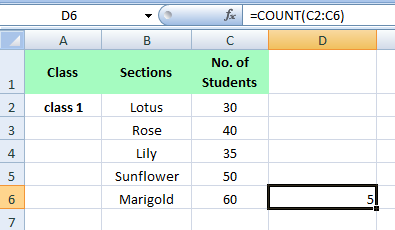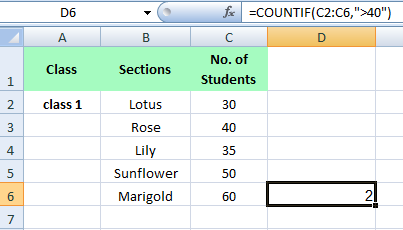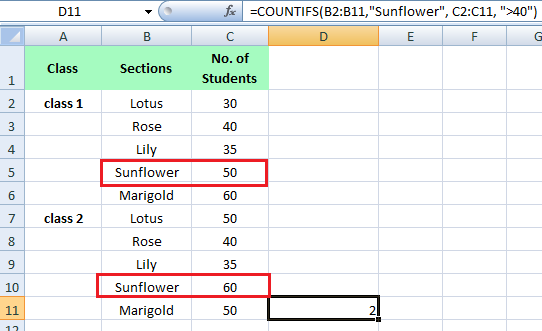### Count Function in MS Excel

The Count function is one of the most used functions in MS Excel. This functions let you count the cells based on one or multiple criteria.

• ### COUNT

• COUNTIF
• COUNTIFS

How to use COUNT function
Let's learn with Example
COUNT function is used to count the number of cells that contain numbers .
D6 equals =COUNT(C2:C6)How to use COUNTIF function
Let's learn with Example
COUNTIF function is used to count cells based on one criteria .
For example, for counting the values greater than 40
D6 equals =COUNTIF(C2:C6,”>40”)How to use COUNTIFS function
Let's learn with Example
COUNTIFS function is used to count cells based on multiple criteria
For example, for counting the values Sunflower and greater than 40
D11 equals =COUNTIFS(B2:B11,”Sunflower”,C2:C11,”>40”)